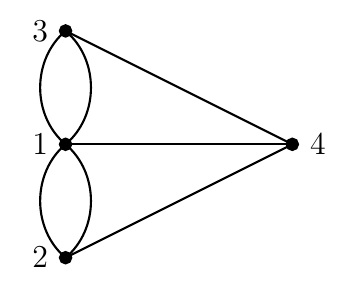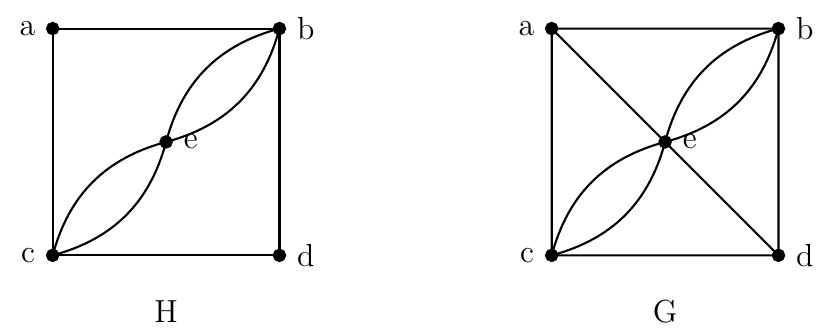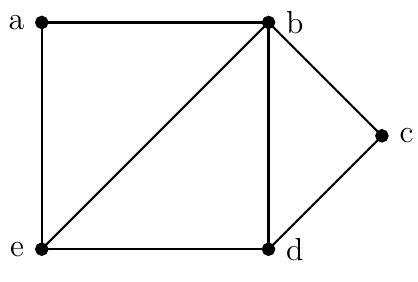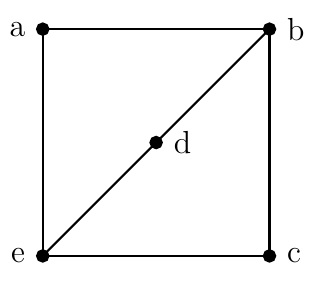Discrete Math Home

## Euler and Hamilton Circuits

Recall the seven bridges of Königsberg problem: Can we cross all the 7 bridges exactly once and come back to the starting point? In other words, is there a circuit in the corresponding graph containing each of its edges exactly once?A graph corresponding to the seven bridges of Königsberg problem

Definition (Euler circuit). An Euler walk in in a connected graph $$G$$ is a walk that visits each edge of $$G$$ exactly once. An Euler circuit in a connected graph $$G$$ is a circuit (i.e., closed walk) that visits each edge of $$G$$ exactly once. A graph $$G$$ is Eulerian if it contains an Euler circuit.

Theorem. A connected graph with at least 2 vertices has an Euler circuit if and only if each of its vertex has even degree.

Theorem. A connected graph with at least 2 vertices has an Euler walk but not a Euler circuit if and only if it has exactly 2 vertices of odd degree.

Example. Determine whether the following graphs have an Euler circuit or an Euler walk.Solution. Since the degree of each vertex of $$H$$ is even, $$H$$ has a Euler circuit, for example, $$abdcebeca$$. Since $$\operatorname{d}(a)=3$$ is odd, $$G$$ has no Euler circuit. Note that $$\operatorname{d}(a)=\operatorname{d}(d)=3$$, $$\operatorname{d}(b)=\operatorname{d}(c)=4$$, and $$\operatorname{d}(e)=6$$. Since $$G$$ has exactly two vertices of odd degree, $$G$$ has an Euler walk from $$a$$ to $$d$$: $$aecebedbacd$$.

Definition (Hamilton circuit). A Hamilton walk in a connected graph $$G$$ is a walk that passes through each vertex of $$G$$ exactly once. A Hamilton circuit in a connected graph $$G$$ is a circuit that passes through each vertex of $$G$$ exactly once.

Example. $$K_2$$ has a Hamilton walk but no Hamilton circuit. $$K_n,n\geq 3$$ has $$\frac{(n-1)!}{2}$$ Hamilton circuits.

Remark. Note that unlike an Euler circuit there are no necessary and sufficient conditions for existence of a Hamilton circuit. But we have some sufficient conditions in terms of enough edges.

Theorem (Dirac's Theorem, 1952). Let $$G$$ be a simple graph on $$n\geq 3$$ vertices. If each vertex of $$G$$ has degree at least $$\frac{n}{2}$$, then $$G$$ has a Hamilton circuit.

Theorem (Ore's Theorem, 1960). Let $$G$$ be a simple graph on $$n\geq 3$$ vertices. If $$\operatorname{d}(u)+\operatorname{d}(v)\geq n$$ for any two non-adjacent vertices $$u$$ and $$v$$ of $$G$$, then $$G$$ has a Hamilton circuit.

Example. The following graph has a Hamilton circuit $$abcdea$$. But it does not satisfy the condition of Dirac's Theorem because $$\operatorname{d}(a)=2\not\geq \frac{5}{2}$$. Also it does not satisfy the condition of Ore's Theorem because $$\operatorname{d}(a)+\operatorname{d}(c)=4\not\geq 5$$.Example. The following graph has no Hamilton circuits. But it has a Hamilton walk $$abced$$.The Travelling Salesman Problem (TSP):
Consider a weighted graph (edges have weights). Find a circuit that passes through each vertex exactly once where the sum of weights of corresponding edges is minimum, i.e., find a Hamilton circuit with minimum weight. This appears in planning, logistics, microchips manufacturing, and DNA sequencing. How hard is it to find a Hamilton circuit? NP-hard (Further reading: computational complexity).

Last edited